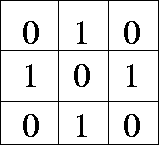Next: References Up: Computer Vision IT412 Previous: Binary Images

# Discrete Binary Images

In a discrete binary image objects are represented in terms of discrete pixels. Calculations of areas, centroids, moments and so on are straightforward -- we use summations instead of the integral equations we used in the continuous case.

Suppose there are several objects in the image. We must separate them before calculating the various moments. We need to establish what makes an object connected or separate from other objects.

Two points in a binary image are connected if a path can be found between them along which the characteristic function remains constant. To decide this we need to know what the topology is, that is, under what conditions do we say that two pixels are neighbours.

There are various possibilities for deciding upon the appropriate topology.

1.
Four connectedness. In this case only edge adjacent pixels are neighbours.
2.
Eight connectedness. In this case edge and corner adjacent cells are considered neighbours.
Neither choice is very good, since neither allow the Jordan Curve Theorem to be satisfied.

The Jordan Curve Theorem says A simple closed curve separates the plane into two simply connected components (namely, the inside and the outside).

Consider the following simple example:If we use 4 connectedness we have 4 separate objects. These objects are not connected so all the background elements should be connected as one object -- but the centre cell (a background one) is not connected to the others, giving us a contradiction. There are (at least) two background regions without a closed curve.

If we use 8 connectedness we have an object that creates a closed curve -- but the background element in the middle is also connected to the outside!

The ideal solution is to use a hexagonal grid, which gives us 6 connectedness. Unfortunately, images don't come in this form.

Another solution is to consider object pixels as 8 connected and background points as 4 connected (or vice versa). However, this asymmetry may be considered undesirable. Alternatively one can skew the rectangular grid.This establishes 6 connectedness. In the undistorted grid this corresponds to an arrangement where the centre pixel is connected to its four immediate neighbours (as in 4-connectedness) plus two of its diagonal neighbours. Thus, one must chose between a left-skewed 6-connectedness, or a right-skewed 6-connectedness.Next: References Up: Computer Vision IT412 Previous: Binary Images
Robyn Owens
10/29/1997